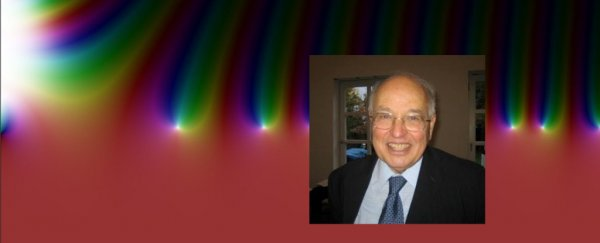A visualisation of the Riemann zeta function, and Sir Michael Atiyah. (Jan Homann/Wikimedia; Gert-Martin Greuel/Wikimedia Commons)

# Top Mathematician Claims He's Solved a \$1 Million Hypothesis. But There's a Problem

WILLIAM ROSS, THE CONVERSATION
30 SEPTEMBER 2018

Over the past few days, the mathematics world has been abuzz over the news that Sir Michael Atiyah, the famous Fields Medalist and Abel Prize winner, claims to have solved the Riemann hypothesis.

If his proof turns out to be correct, this would be one of the most important mathematical achievements in many years.

In fact, this would be one of the biggest results in mathematics, comparable to the proof of Fermat's Last Theorem from 1994 and the proof of the Poincare Conjecture from 2002.

Besides being one of the great unsolved problems in mathematics and therefore garnishing glory for the person who solves it, the Riemann hypothesis is one of the Clay Mathematics Institute's "Million Dollar Problems." A solution would certainly yield a pretty profitable haul: one million dollars.

The Riemann hypothesis has to do with the distribution of the prime numbers, those integers that can be divided only by themselves and one, like 3, 5, 7, 11 and so on.

We know from the Greeks that there are infinitely many primes. What we don't know is how they are distributed within the integers.

The problem originated in estimating the so-called "prime pi" function, an equation to find the number of primes less than a given number.

But its modern reformulation, by German mathematician Bernhard Riemann in 1858, has to do with the location of the zeros of what is now known as the Riemann zeta function.

The technical statement of the Riemann hypothesis is "the zeros of the Riemann zeta function which lie in the critical strip must lie on the critical line."

Even understanding that statement involves graduate-level mathematics courses in complex analysis.

Most mathematicians believe that the Riemann hypothesis is indeed true. Calculations so far have not yielded any misbehaving zeros that do not lie in the critical line.

However, there are infinitely many of these zeros to check, and so a computer calculation will not verify all that much. Only an abstract proof will do.

If, in fact, the Riemann hypothesis were not true, then mathematicians' current thinking about the distribution of the prime numbers would be way off, and we would need to seriously rethink the primes.

The Riemann hypothesis has been examined for over a century and a half by some of the greatest names in mathematics and is not the sort of problem that an inexperienced math student can play around with in his or her spare time.

Attempts at verifying it involve many very deep tools from complex analysis and are usually very serious ones done by some of the best names in mathematics.

Atiyah gave a lecture in Germany on September 25 in which he presented an outline of his approach to verify the Riemann hypothesis. This outline is often the first announcement of the solution but should not be taken that the problem has been solved – far from it.

For mathematicians like me, the "proof is in the pudding," and there are many steps that need to be taken before the community will pronounce Atiyah's solution as correct.

First, he will have to circulate a manuscript detailing his solution. Then, there is the painstaking task of verifying his proof. This could take quite a lot of time, maybe months or even years.

Is Atiyah's attempt at the Riemann hypothesis serious? Perhaps.

His reputation is stellar, and he is certainly capable enough to pull it off. On the other hand, there have been several other serious attempts at this problem that did not pan out.

At some point, Atiyah will need to circulate a manuscript that experts can check with a fine-tooth comb.William Ross, Professor of Mathematics, University of Richmond.